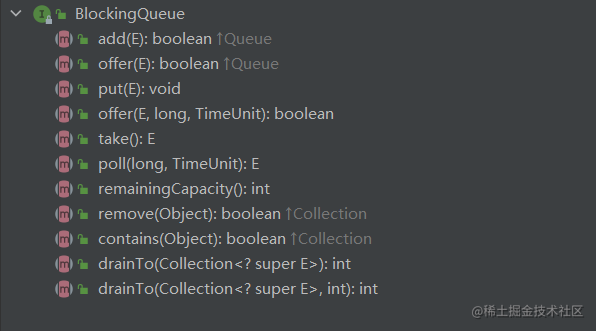# 数据结构与算法 | 队列与栈的经典操作以及Java中的List和Queue

### 用数组实现栈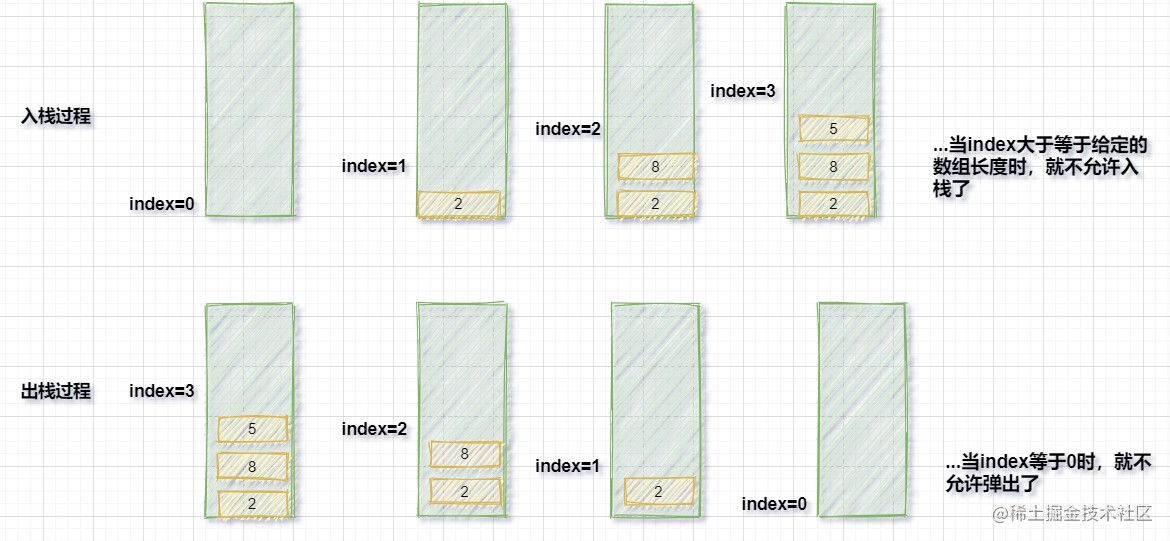``````public class MyStack {
private int[] array;
private int index;

public MyStack(int size) {
this.array = new int[size];
}

//入栈
public void push(int value) {
if (index >= array.length) {
throw new RuntimeException("栈满，不让加了");
}
array[index++] = value;
}

//出栈
public int pop() {
if (index <= 0) {
throw new RuntimeException("栈空，不能取出");
}
return array[--index];
}
}
``````

### 用数组实现队列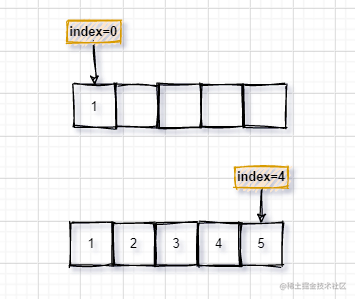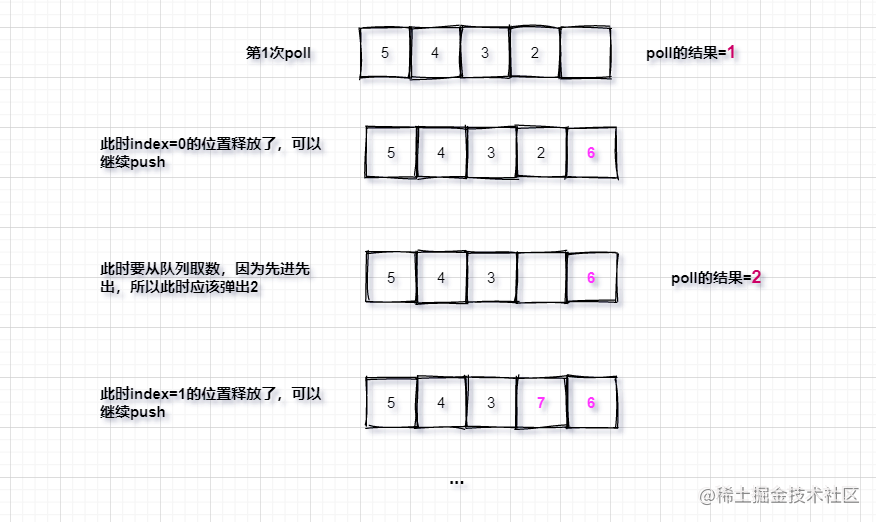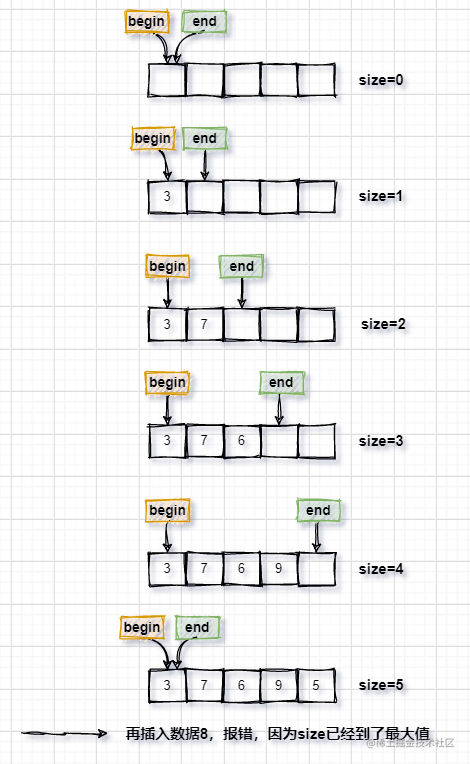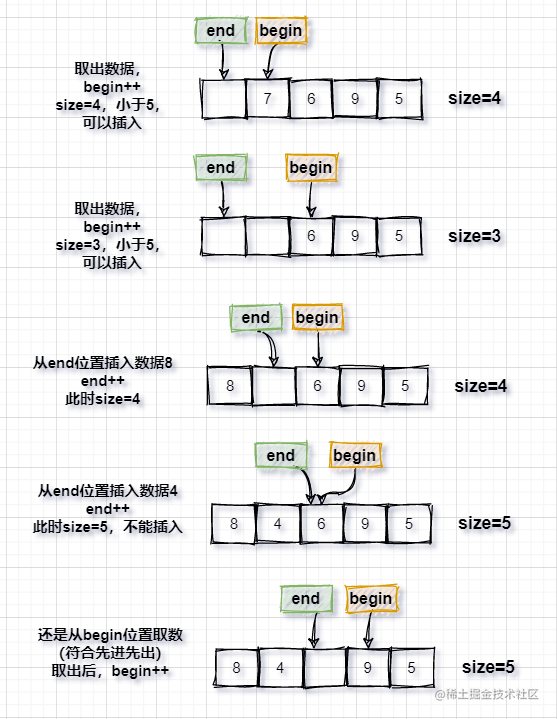``````public static class MyQueue {
private int[] array;
private int begin;
private int end;
private int size;

public MyQueue (int limit) {
this.array = new int[limit];
this.begin = 0;
this.end = 0;
this.size = 0;
}

public void push (int value) {
size++;
if (size > array.length) {
throw new RuntimeException("队列满了");
}
array[end] = value;
end++;
//针对end越界的处理
if (end >= array.length) {
end = 0;
}
}

public int pop () {
size--;
if (size < 0) {
throw new RuntimeException("队列已空");
}
int result = array[begin];
begin++;
//针对begin越界的处理
if (begin >= array.length) {
begin = 0;
}
return result;
}
}
``````

### 一些经典操作

• 用栈结构实现队列结构
• 用队列结构实现栈结构

#### 用栈实现队列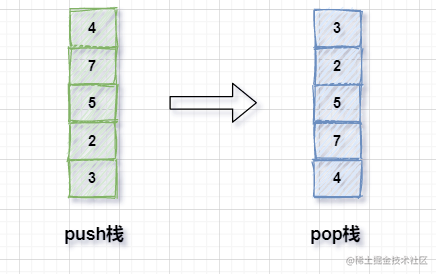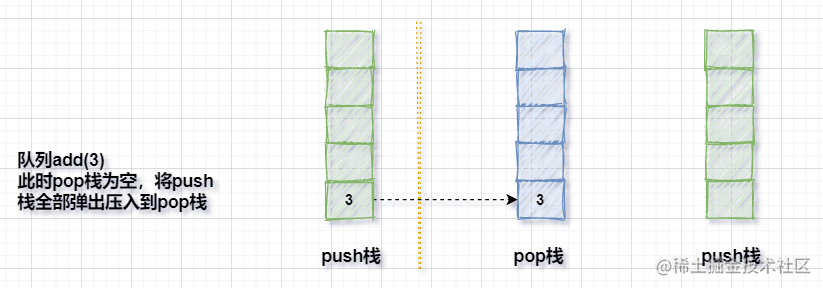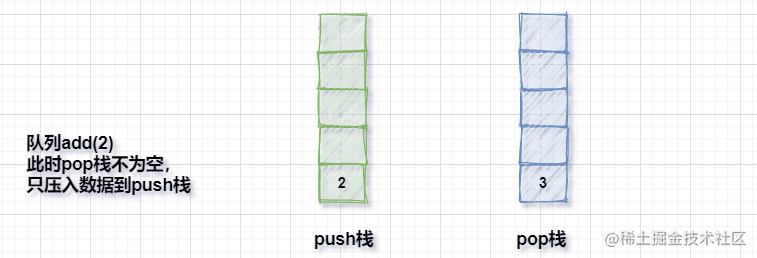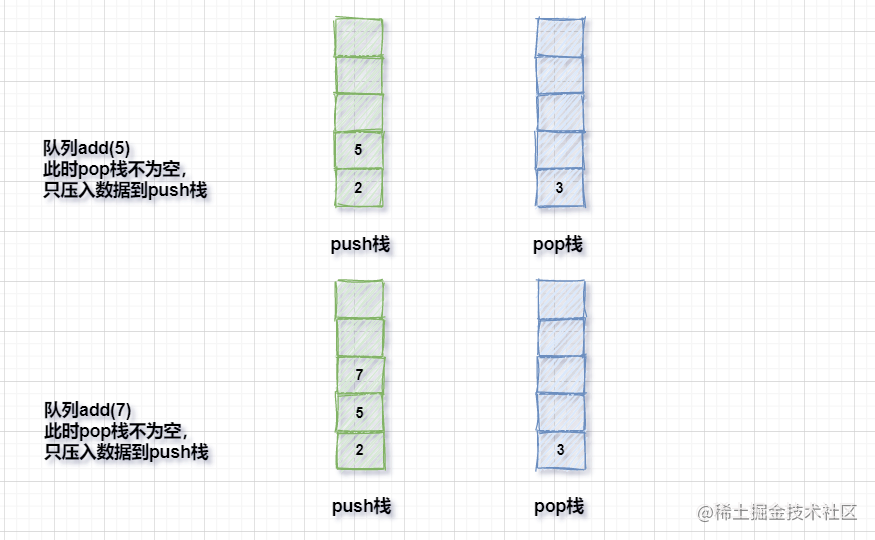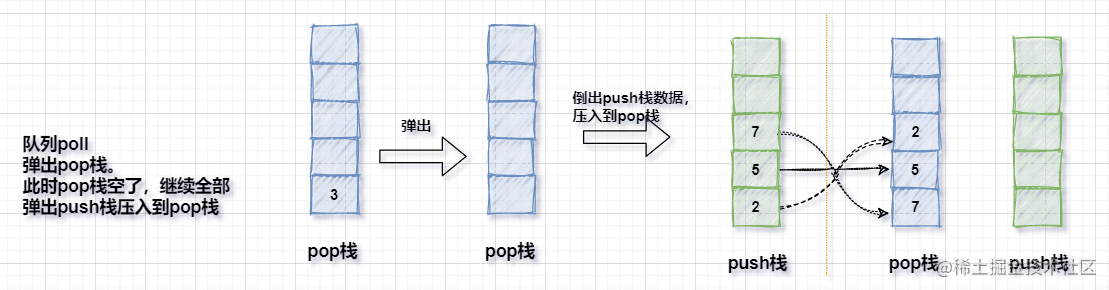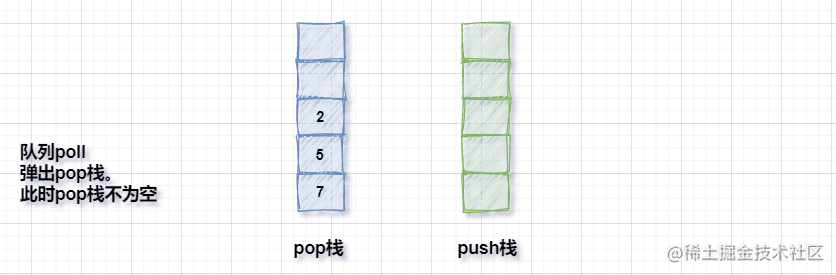• push栈数倒入到pop栈时要一次性倒完
• 当pop栈不为空时，不需要压入push栈的数据

``````public void pushToPop() {
if (popStack.isEmpty()) {
while (!pushStack.isEmpty()) {
popStack.push(pushStack.pop());
}
}
}
``````

``````public class MyQueueWithStack {
private Stack<Integer> pushStack;
private Stack<Integer> popStack;

public MyQueueWithStack() {
this.pushStack = new Stack<>();
this.popStack = new Stack<>();
}

public void pushToPop() {
if (popStack.isEmpty()) {
while (!pushStack.isEmpty()) {
popStack.push(pushStack.pop());
}
}
}

pushStack.push(value);
pushToPop();
}

public int poll() {
if (popStack.isEmpty() && pushStack.isEmpty()) {
throw new RuntimeException("队列空了！");
}
pushToPop();
return popStack.pop();
}
}
``````

#### 用队列实现栈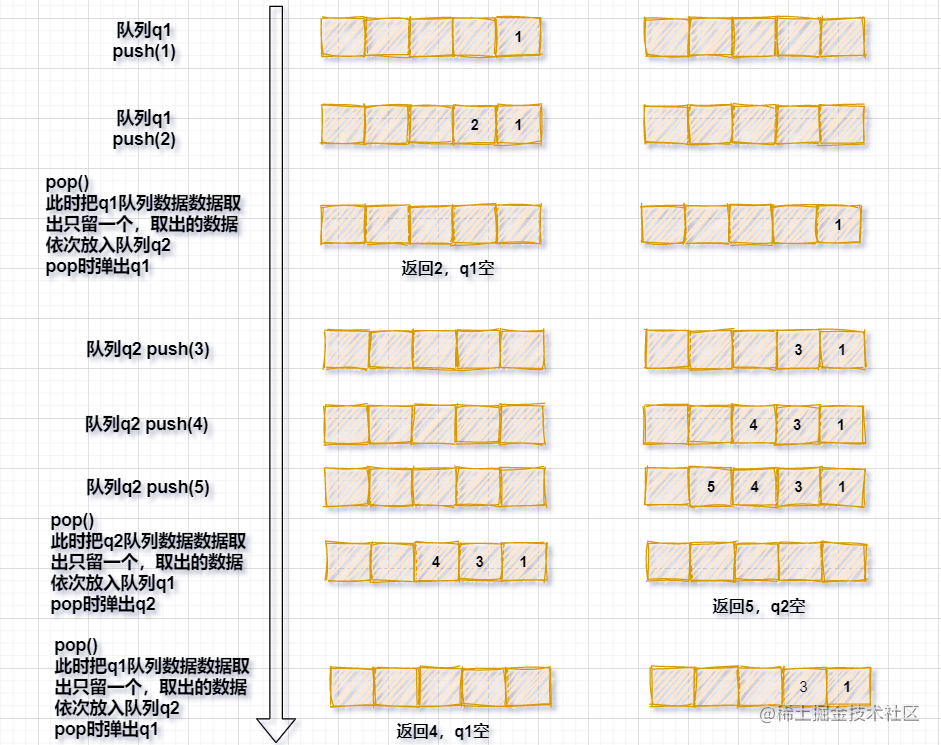...

``````public class MyStackWithQueue<T> {
private Queue<T> queue;
private Queue<T> help;

public MyStackWithQueue() {
}

public void push(T value) {
if (queue.isEmpty() && help.isEmpty()) {
}
if (!queue.isEmpty()) {
}
if (!help.isEmpty()) {
}
}

public T pop() {
if (!queue.isEmpty()) {
temp = queue;
while (queue.size() > 1) {
}
} else if (!help.isEmpty()) {
temp = help;
while (help.size() > 1) {
}
}
return temp.poll();
}
}
``````

### 延伸：Java中的List和Queue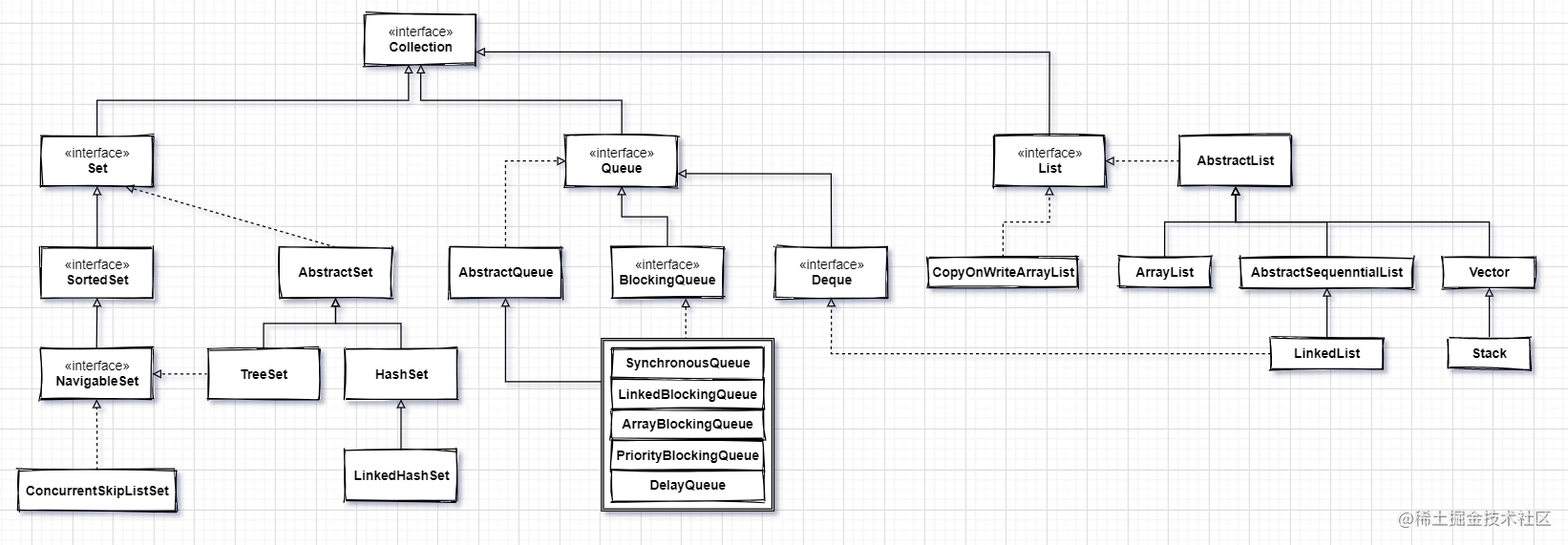#### List集合

List集合元素有明确的 上一个下一个 元素，也存在明确的第一个和最后一个元素。

##### ArrayList

ArrayList的容量可以改变，非线程安全集合。其内部实现用数组进行存储，集合扩容时会创建一个更大的数组控件，把原有数据复制到新数组中。

``````public class ArrayList<E> extends AbstractList<E>
implements List<E>, RandomAccess, Cloneable, java.io.Serializable
{
private static final long serialVersionUID = 8683452581122892189L;

/**
* Default initial capacity.
*/
private static final int DEFAULT_CAPACITY = 10;

/**
* Shared empty array instance used for empty instances.
*/
private static final Object[] EMPTY_ELEMENTDATA = {};

/**
* Shared empty array instance used for default sized empty instances. We
* distinguish this from EMPTY_ELEMENTDATA to know how much to inflate when
*/
private static final Object[] DEFAULTCAPACITY_EMPTY_ELEMENTDATA = {};

/**
* The array buffer into which the elements of the ArrayList are stored.
* The capacity of the ArrayList is the length of this array buffer. Any
* empty ArrayList with elementData == DEFAULTCAPACITY_EMPTY_ELEMENTDATA
* will be expanded to DEFAULT_CAPACITY when the first element is added.
*/
transient Object[] elementData; // non-private to simplify nested class access

//......
}
``````

ArrayList支持对元素的快速随机访问，但是插入和删除的速度较慢，因为插入和删除的过程需要移动元素。

``````public class LinkedList<E>
extends AbstractSequentialList<E>
implements List<E>, Deque<E>, Cloneable, java.io.Serializable
{
transient int size = 0;

/**
* Pointer to first node.
* Invariant: (first == null && last == null) ||
*            (first.prev == null && first.item != null)
*/
transient Node<E> first;

/**
* Pointer to last node.
* Invariant: (first == null && last == null) ||
*            (last.next == null && last.item != null)
*/
transient Node<E> last;

//...
}
``````

#### Queue集合